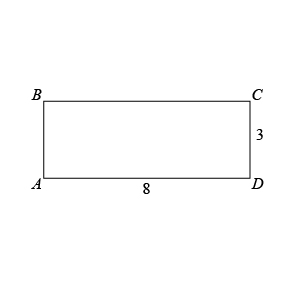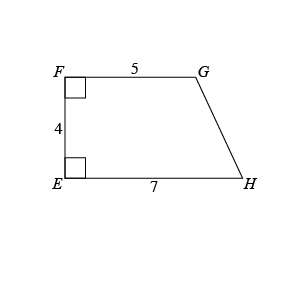# The area of rectangular region ABCD The area of trapezoidal regionQuantity A Quantity B The area of rectangular region \$ABCD\$ The area of trapezoidal region \$EFGH\$
1. Quantity A is greater.
2. Quantity B is greater.
3. The two quantities are equal.
4. The relationship cannot be determined from the information given

So, you were trying to be a good test taker and practice for the GRE with PowerPrep online. Buuuut then you had some questions about the quant section—specifically question 3 of Section 4 of Practice Test 1. Those Quadrilateral questions can be kind of tricky, but never fear, PrepScholar has got your back!

## Survey the Question

Let’s search the problem for clues as to what it will be testing, as this will help shift our minds to think about what type of math knowledge we’ll use to solve this question. Pay attention to any words that sound math-specific and anything special about what the numbers look like, and mark them on your paper.

The question has two \$4\$-sided figures. We can expect that it tests our knowledge of Quadrilaterals from geometry.

## What Do We Know?

Let’s carefully read through the question and make a list of the things that we know.

1. We’re given a rectangle, along with the lengths of its sides
2. We’re given a trapezoid, along with the lengths of its bases and height
3. We want to compare the area of the square to the area of the trapezoid

## Develop a Plan

Geometry! Well, at least they were kind enough to give us pictures to play with. So we can skip the valuable step of creating figures for most geometry questions. However, we should feel free to quickly redraw these figures on our scrap paper, as it’s hard to write on the computer test screen (and frowned upon by the test proctors).

Let’s start with a top-down approach, where we will begin with what we’re looking for and work down to the details of what we’re given in this question. Starting with Quantity A, we want to know the area of rectangle \$ABCD\$. Thinking of equations for areas of quadrilaterals, we do know that:

\$\$\Area \Rectangle = \Length · \Width\$\$

We see that rectangle \$ABCD\$ has a length and width of \$3\$ and \$8\$, so we definitely have enough information to calculate its area.

Moving onto Quantity B. We need the area of trapezoid \$EFGH\$. Ah, we learned this when we learned about areas of quadrilaterals.

\$\$\Area \Trapezoid = (\Base 1 + \Base 2)/2·\Height\$\$

In trapezoids, the two bases must be parallel to each other, and the height is the side perpendicular (forming a \$90°\$ angle) to both of the bases. Using this info, we can see that the two bases have lengths of \$5\$ and \$7\$, respectively, and the height has a length of \$4\$.

Now we have enough information to solve for both areas, so let’s get to it!

## Solve the Question

First, solving for Quantity A we get:

 \$\Area \Rectangle\$ \$=\$ \$\Length · \Width\$ \$\Area \Rectangle\$ \$=\$ \$3·8\$ \$\Area \Rectangle\$ \$=\$ \$24\$

So Quantity A, the area of the rectangle, has the value \$24\$. Now let’s solve for Quantity B, the area of the trapezoid.

 \$\Area \Trapezoid\$ \$=\$ \$(\Base 1 + \Base 2)/2·\Height\$ \$\Area \Trapezoid\$ \$=\$ \$(5+7)/2·4\$ \$\Area \Trapezoid\$ \$=\$ \$12/2·4\$ \$\Area \Trapezoid\$ \$=\$ \$24\$

Ah! So both quantities have the value of \$24\$, so they are equal to each other.

The correct answer is C, the two quantities are equal.

## What Did We Learn

While we likely knew the equation for the area of a square, the equation for the area of a trapezoid is a little less well-known:

\$\$\Area \of \Trapezoid = (\Base 1 + \Base 2)/2·\Height\$\$

In a trapezoid, let’s remember that the two bases are parallel to each other, and the height is the side that is perpendicular to both bases. Let’s commit this equation to memory so that we can slay any trapezoid questions on test day with ease!

Want more expert GRE prep? Sign up for the five-day free trial of our PrepScholar GRE Online Prep Program to access your personalized study plan with 90 interactive lessons and over 1600 GRE questions.